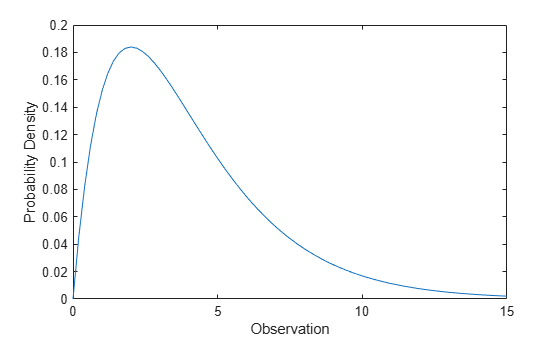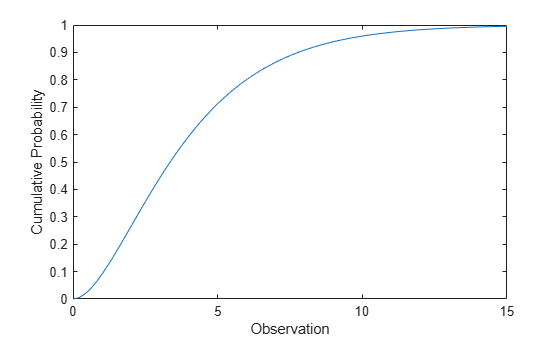## Chi-Square Distribution

### Overview

The chi-square (χ2) distribution is a one-parameter family of curves. The chi-square distribution is commonly used in hypothesis testing, particularly the chi-square test for goodness of fit.

Statistics and Machine Learning Toolbox™ offers multiple ways to work with the chi-square distribution.

### Parameters

The chi-square distribution uses the following parameter.

ParameterDescriptionSupport
nu (ν)Degrees of freedomν` = 1, 2, 3,...`

The degrees of freedom parameter is typically an integer, but chi-square functions accept any positive value.

The sum of two chi-square random variables with degrees of freedom ν1 and ν2 is a chi-square random variable with degrees of freedom ν = ν1 + ν2.

### Probability Density Function

The probability density function (pdf) of the chi-square distribution is

`$y=f\left(x|\nu \right)=\frac{{x}^{\left(\nu -2\right)/2}{e}^{-x/2}}{{2}^{\frac{\nu }{2}}\Gamma \left(\nu /2\right)},$`

where ν is the degrees of freedom and Γ( · ) is the Gamma function.

For an example, see Compute Chi-Square Distribution pdf.

### Cumulative Distribution Function

The cumulative distribution function (cdf) of the chi-square distribution is

`$p=F\left(x|\nu \right)={\int }_{0}^{x}\frac{{t}^{\left(\nu -2\right)/2}{e}^{-t/2}}{{2}^{\nu /2}\Gamma \left(\nu /2\right)}dt,$`

where ν is the degrees of freedom and Γ( · ) is the Gamma function. The result p is the probability that a single observation from the chi-square distribution with ν degrees of freedom falls in the interval [0, x].

For an example, see Compute Chi-Square Distribution cdf.

### Inverse Cumulative Distribution Function

The inverse cumulative distribution function (icdf) of the chi-square distribution is

`$x={F}^{-1}\left(p|\nu \right)=\left\{x:F\left(x|\nu \right)=p\right\},$`

where

`$p=F\left(x|\nu \right)={\int }_{0}^{x}\frac{{t}^{\left(\nu -2\right)/2}{e}^{-t/2}}{{2}^{\nu /2}\Gamma \left(\nu /2\right)}dt,$`

ν is the degrees of freedom, and Γ( · ) is the Gamma function. The result p is the probability that a single observation from the chi-square distribution with ν degrees of freedom falls in the interval [0, x].

### Descriptive Statistics

The mean of the chi-square distribution is ν.

The variance of the chi-square distribution is 2ν.

### Examples

#### Compute Chi-Square Distribution pdf

Compute the pdf of a chi-square distribution with 4 degrees of freedom.

```x = 0:0.2:15; y = chi2pdf(x,4);```

Plot the pdf.

```figure; plot(x,y) xlabel('Observation') ylabel('Probability Density')```The chi-square distribution is skewed to the right, especially for few degrees of freedom.

#### Compute Chi-Square Distribution cdf

Compute the cdf of a chi-square distribution with 4 degrees of freedom.

```x = 0:0.2:15; y = chi2cdf(x,4);```

Plot the cdf.

```figure; plot(x,y) xlabel('Observation') ylabel('Cumulative Probability')```### Related Distributions

• F Distribution — The F distribution is a two-parameter distribution that has parameters ν1 (numerator degrees of freedom) and ν2 (denominator degrees of freedom). The F distribution can be defined as the ratio $F=\frac{{\chi }_{1}^{2}}{{\nu }_{1}}}{{\chi }_{2}^{2}}{{\nu }_{2}}}$, where χ21 and χ22 are both chi-square distributed with ν1 and ν2 degrees of freedom, respectively.

• Gamma Distribution — The gamma distribution is a two-parameter continuous distribution that has parameters a (shape) and b (scale). The chi-square distribution is equal to the gamma distribution with 2a = ν and b = 2.

• Noncentral Chi-Square Distribution — The noncentral chi-square distribution is a two-parameter continuous distribution that has parameters ν (degrees of freedom) and δ (noncentrality). The noncentral chi-square distribution is equal to the chi-square distribution when δ = 0.

• Normal Distribution — The normal distribution is a two-parameter continuous distribution that has parameters μ (mean) and σ (standard deviation). The standard normal distribution occurs when μ = 0 and σ = 1.

If Z1, Z2, …, Zn are standard normal random variables, then $\sum _{i=1}^{n}{Z}_{i}{}^{2}$ has a chi-square distribution with degrees of freedom ν = n – 1.

If a set of n observations is normally distributed with variance σ2 and sample variance s2, then $\frac{\left(n-1\right){s}^{2}}{{\sigma }^{2}}$ has a chi-square distribution with degrees of freedom ν = n – 1. This relationship is used to calculate confidence intervals for the estimate of the normal parameter σ2 in the function `normfit`.

• Student's t Distribution — The Student's t distribution is a one-parameter continuous distribution that has parameter ν (degrees of freedom). If Z has a standard normal distribution and χ2 has a chi-square distribution with degrees of freedom ν, then has a Student's t distribution with degrees of freedom ν.

• Wishart Distribution — The Wishart distribution is a higher dimensional analog of the chi-square distribution.

 Abramowitz, Milton, and Irene A. Stegun, eds. Handbook of Mathematical Functions: With Formulas, Graphs, and Mathematical Tables. 9. Dover print.; [Nachdr. der Ausg. von 1972]. Dover Books on Mathematics. New York, NY: Dover Publ, 2013.

 Devroye, Luc. Non-Uniform Random Variate Generation. New York, NY: Springer New York, 1986. https://doi.org/10.1007/978-1-4613-8643-8

 Evans, M., N. Hastings, and B. Peacock. Statistical Distributions. 2nd ed., Hoboken, NJ: John Wiley & Sons, Inc., 1993.

 Kreyszig, Erwin. Introductory Mathematical Statistics: Principles and Methods. New York: Wiley, 1970.